## Runge Kutta-2

Suppose we have ODE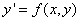then

Formula :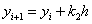Where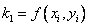and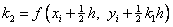Other variation name : Improve Euler method, Heun's method, Midpoint method

Error term :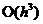, correct up to the second order term in Taylor series expansion.

The Taylor series expansion is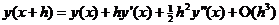Example:, set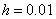, with initial condition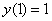. The few first results and the graph of solution are given below.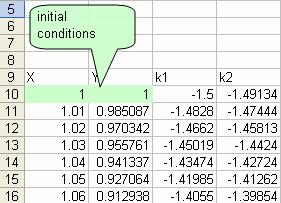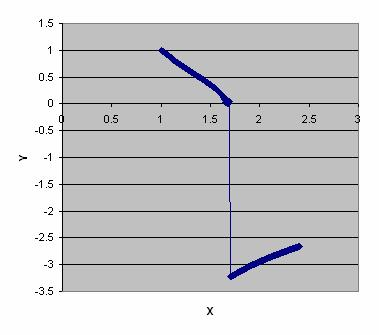Note that after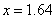, the solution is not correct. See Comparison.

Expanding the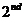order Runge-Kutta formula, we have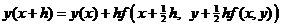Second term of the right hand side [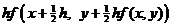] is the estimated range difference. The estimated range difference is computed based on a half of the slope at the beginning of the interval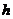and the slope in the middle of the interval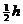, as illustrated in the figure below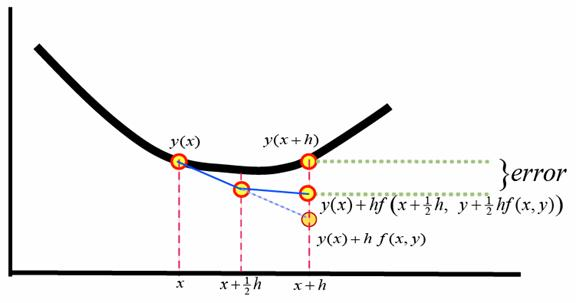Preferable reference for this tutorial is

Teknomo, Kardi (2015) Solving Ordinary Differential Equation (ODE). https:\\people.revoledu.com\kardi\ tutorial\ODE\